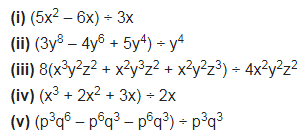# Divide the given polynomial by the given monomial, (i) (5x2 – 6x) ÷ 3x

Divide the given polynomial by the given monomial,